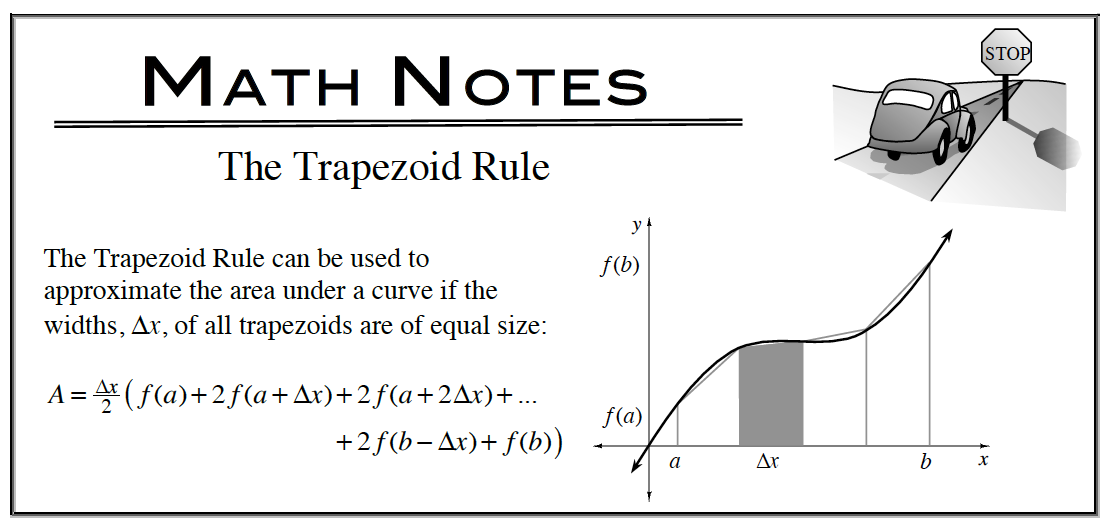### Home > APCALC > Chapter 5 > Lesson 5.4.1 > Problem5-147

5-147.

A function f is continuous over the interval [2, 8] and has values that are given in the table at right. Homework Help ✎

1. Using the sub-intervals [2, 5], [5, 7], and [7, 8], what is the trapezoidal approximation of $\int _ { 2 } ^ { 8 } f ( x ) d x$?2. Over which sub-interval is the average slope a minimum?

 I. [2, 5] II. [5, 7] III. [7, 8]

Test all answer choices and choose the lowest value. Recall that average slope is akin to saying average rate of change (AROC).

 x f (x) 2 10 5 30 7 40 8 20